## sclduggery

Here’s a picture of a once-punctured genus 1 surface.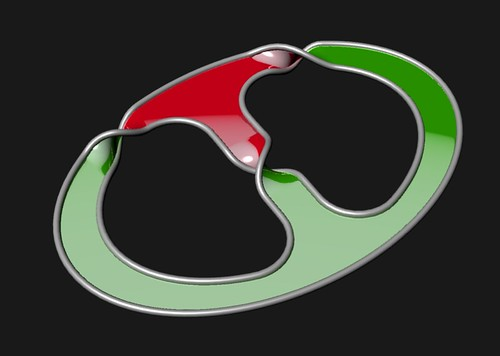You can put it in a genus two handlebody. View the handlebody as corresponding to the free group on two generators$a$ and$b$. Here I’m showing them as the yellow and blue cores of two handles.

Then the boundary of this once-punctured genus 1 surface may be viewed as representing an element of this free group.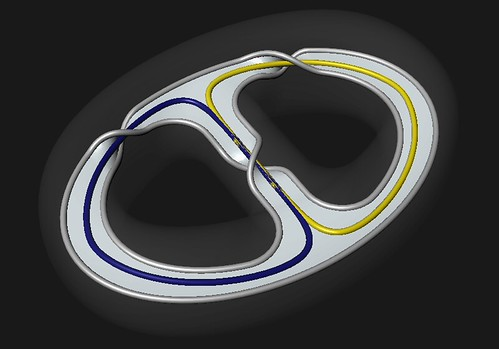Using the correspondence we can write the boundary of the surface as the product$a b a^{-1} b^{-1}$ which is also denoted$[a,b]$ and known as the commutator of$a$ and$b$.

Those of y’all who have learned a bit about fundamental groups know that the boundary of a (compact, orientable) once-punctured genus k surface can be expressed as the product of$k$ commutators of curves on the surface. Y’all also know that I’m being loose with basepoints, curves, and group elements.

At the Georgia Topology Fest this past May, Calegari spoke about scl, where this mix of the algebra and geometry of this can lead. He discusses it in greater detail in a recent entry of his blog. I’ll tell you a bit and then show off a fun fundamental example.

The elements of the commutator subgroup$[G,G]$ of a group$G$ can all be written as a product of commutators. That is to say if$c \in [G,G]$ then there exists a finitely many (say,$n$) elements$a_i, b_i \in G$ such that$c = \Pi_1^n [a_i, b_i] = \Pi_1^n (a_i b_i a_i^{-1} b_i^{-1}) = a_1 b_1 a_1^{-1} b_1^{-1} a_2 b_2 a_2^{-1} b_2^{-1} \dots a_n b_n a_n^{-1} b_n^{-1}$.

The commutator length, or simply cl, of a given$c \in [G,G]$ is just the smallest number (the smallest$n$) of commutators (the$[a_i,b_i]$) needed to express$c$ as their product.

The stable commutator length, aka scl, of a given$c \in [G,G]$ reflects the asymptotic behavior of$\frac{1}{k}$cl$(c^k)$ as$k$ grows.

One’s first impulse is to say that$\frac{1}{k}$cl$(c^k)$ is just cl$(c)$. Take the example at the very top. Of course$[a,b]^3 = [a,b][a,b][a,b]$, but isn’t it obvious that you can’t do any better?

Here’s a few pictures even.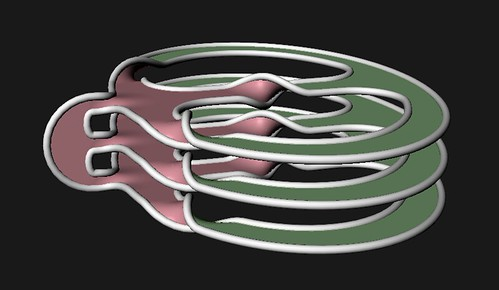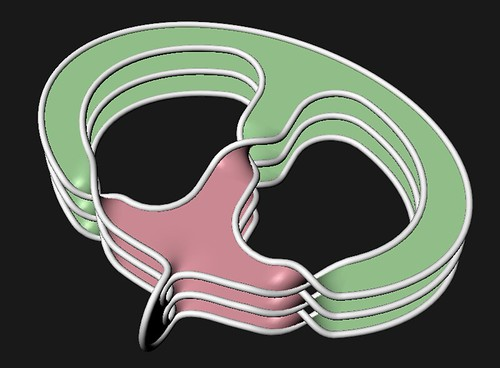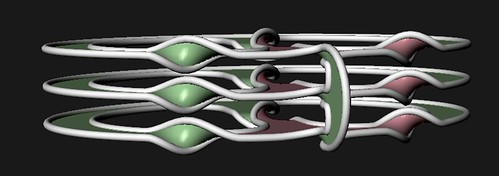Go ahead. See if you can do better.
.

.

.

Don’t wanna spoil the fun.

.

.

.

Here’s a monkey washing a cat.

.

.

.

That always gets my head a thinkin’.

.

.

.

Yeah, so if by “obvious” you meant “wrong”, then you are correct.

.

.

.

Thinking about Euler characteristics and irregular three-fold covers leads you to a genus 2 surface.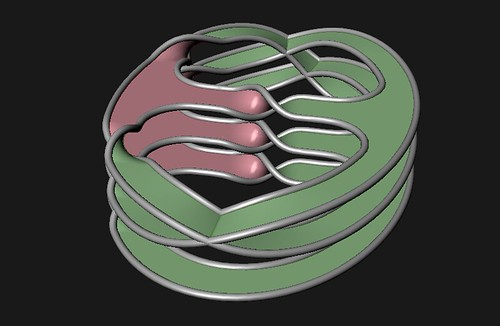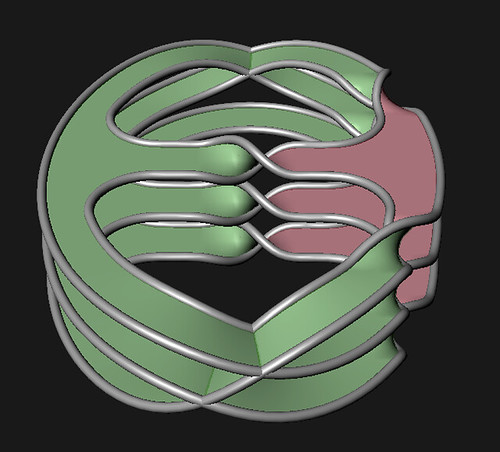So cl$([a,b]^3) = 2$. (Why is it not 1?) Hence$\frac{1}{3}$cl$([a,b]^3) = \frac{2}{3}$

~ by Ken Baker on July 24, 2009.

### One Response to “sclduggery”

1. […] If then the loops representing and can be thought of as the meridian and the longitude of the bounding torus. There’s some very nice pictures of this (and loads of other stuff) at the blog Sketches of Topology. […]